## Example Questions

2 Next →

### Example Question #11 : Solid Geometry

Find the surface area of a cube with side length 2.Explanation:

To solve, simply use the formula for the surface area of a cube. Thus,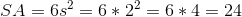### Example Question #711 : Geometry

Find the surface area of a cube given side length of 3.Explanation:

To find the surface area of a cube means to find the area around the entire object. In the case of a cube, we will need to find that area of all the sides and the top and bottom. Since a cube has equal side lengths, the area of each side and the area of the top and bottom will all be the same.

Recall that the area for a side of a cube is: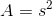From here there are two approaches one can take.

Approach one: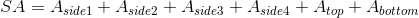Approach two:

Use the formula for the surface area of a cube,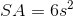In this particular case we are given the side length is 3.

Thus we can find the surface area to be,

by approach one,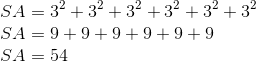and by appraoch two,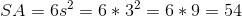.

### Example Question #22 : Cubes

Find the volume of a cube given side length of 3.Explanation:

To solve, simply use the formula for the surface area of a cube.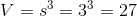If you do not remember the formula for the test, it is important to draw a picture or to visually conceptualize it. Remember, when finding area of a square or rectangle, you simply multiply the two side lengths. So for a cube in 3 dimensions, you simply have to multiply those three together.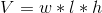However, a cube is a special case where all three lengths are the same. Thus,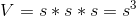### Example Question #711 : Sat Mathematics

A cube has given volume. What is its surface area?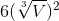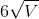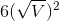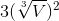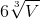Explanation:

First, we need the side length of the cube. Given that, whereis a side length, we can solve forand set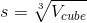simply by taking the cube root of both sides.

Then we need to remember or realize that the surface area of a cube is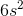because there will be six identical square faces. Plugging in, we getas our answer.

### Example Question #12 : How To Find The Surface Area Of A Cube

Find the surface area of a cube with side length 8.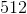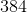Explanation:

To solve, simply use the formula for the surface area of a cube.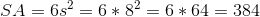If this is not a formula you have committed to memory, remember that a cube has 6 faces with equal area. So, start by calculating the surface area of one side (64) and add it 6 times. Thus,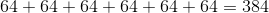### Example Question #721 : Geometry

You own a Rubik's cube with a volume of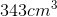. What is the surface area of the cube?

Not enough information to solve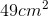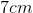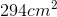Explanation:

You own a Rubik's cube with a volume of. What is the surface area of the cube?

To solve for edge length, think of the volume of a cube formula: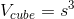Now, we have the volume, so just rearrange it to solve for side length: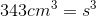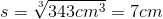Next, recall the surface area of a cube formula: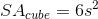Plug in and simplify to get: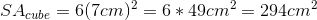### Example Question #591 : Problem Solving

A room has dimensions of 23ft by 17ft by 10ft. The last dimension is the height of the room. It has one door that is 2.5ft by 8ft and one window, 3ft by 6ft. There is no trim to the floor, wall, doors, or windows. If one can of paint covers 57 ft2 of surface area. How many cans of paint must be bought to paint the walls of the room.

11

15

13

14

18

14

Explanation:

If broken down into parts, this is an easy problem. It is first necessary to isolate the dimensions of the walls. If the room is 10ft high, we know 23 x 17 designates the area of the floor and ceiling. Based on this, we know that the room has the following dimensions for the walls: 23 x 10 and 17 x 10. Since there are two of each, we can calculate the total area of walls - ignoring doors and windows - by doubling the sum of these two areas:

2 * (23 * 10 + 17 * 10) = 2 * (230 + 170) = 2 * 400 = 800 ft2

Now, we merely need to calculate the area "taken out" of the walls:

For the door: 2.5 * 8 = 20 ft2

For the windows: 3 * 6 = 18 ft2

The total wall space is therefore: 800 – 20 – 18 = 762 ft2

Now, if one can of paint covers 57 ft2, we calculate the number of cans necessary by dividing the total exposed area by 57: 762/57 = (approx.) 13.37.

Since we cannot buy partial cans, we must purchase 14 cans.

2 Next →

### All SAT Math Resources